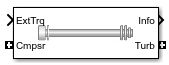Documentation

# Boost Drive Shaft

Boost drive shaft speed

• Library:
• Powertrain Blockset / Propulsion / Combustion Engine Components / Boost## Description

The Boost Drive Shaft block uses the compressor, turbine, and external torques to calculate the drive shaft speed. Use the block to model turbochargers and superchargers in an engine model.

You can specify these configurations:

• Turbocharger — Connect the compressor to the turbine

• Two-way ports for turbine and compressor connections

• Option to add an externally applied input torque

• Compressor only — Connect the drive shaft to the compressor

• Two-way port for compressor connection

• Externally applied input torque

• Turbine only — Connect the drive shaft to the turbine

• Two-way port for turbine connection

• Externally applied load torque

For the `Turbine only` and `Turbocharger` configurations, the block modifies the turbine torque with a mechanical efficiency.

### Equations

The Boost Drive Shaft block applies Newton’s Second Law for Rotation. Positive torques cause the drive shaft to accelerate. Negative torques impose a load and decelerate the drive shaft.

The block also calculates the power loss due to mechanical inefficiency.

CalculationEquations
Shaft dynamics$\frac{d\omega }{dt}=\frac{1}{{J}_{shaft}}\left({\eta }_{mech}{\tau }_{turb}+{\tau }_{comp}+{\tau }_{ext}\right)$ with initial speed ω0

Speed constraint

${\omega }_{min}\le \omega \le {\omega }_{max}$

Power loss

${\stackrel{˙}{W}}_{loss}=\omega {\tau }_{turb}\left(1-{\eta }_{mech}\right)$

### Power Accounting

For the power accounting, the block implements these equations.

Bus Signal DescriptionEquations

`PwrInfo`

`PwrTrnsfrd` — Power transferred between blocks

• Positive signals indicate flow into block

• Negative signals indicate flow out of block

`PwrCmpsr`

Shaft power from compressor

${\tau }_{comp}\omega$

`PwrTurb`

Shaft power from turbine

${\tau }_{turb}\omega$

`PwrExt`Externally applied power

${\tau }_{ext}\omega$

`PwrNotTrnsfrd` — Power crossing the block boundary, but not transferred

• Positive signals indicate an input

• Negative signals indicate a loss

`PwrMechLoss`Mechanical power loss

$-{\stackrel{˙}{W}}_{turb}$

`PwrStored` — Stored energy rate of change

• Positive signals indicate an increase

• Negative signals indicate a decrease

`PwrStoredDriveshft`Rate change in rotational kinetic energy

$\left({\eta }_{mech}{\tau }_{turb}+{\tau }_{comp}+{\tau }_{ext}\right)\omega$

The equations use these variables.

 ω Shaft speed ω0 Initial drive shaft speed ωmin Minimum drive shaft speed ωmax Maximum drive shaft speed Jshaft Shaft inertia ηmax Mechanical efficiency of turbine τcomp Compressor torque τturb Turbine torque τext Externally applied torque. ${\stackrel{˙}{W}}_{loss}$ Power loss due to mechanical inefficiency

## Ports

### Input

expand all

Compressor torque, τcomp, in N·m.

#### Dependencies

To create this port, for the Configuration parameter, select `Turbocharger` or `Compressor only`.

Turbine torque, τturb, in N·m.

#### Dependencies

To create this port, for the Configuration parameter, select `Turbocharger` or ```Turbine only```.

Externally applied torque, τext, in N·m.

#### Dependencies

For turbocharger configurations, to create this port, set Additional torque input to `External torque input`.

### Output

expand all

Bus signal containing these block calculations.

SignalDescriptionUnits

`DriveshftSpd`

Shaft speed

`MechPwrLoss`

Mechanical power loss

W

`ExtTrq`

Applied external torque

N·m
`PwrInfo``PwrTrnsfrd``PwrCmpsr`

Shaft power from compressor

W

`PwrTurb`

Shaft power from turbine

W
`PwrExt`Externally applied powerW
`PwrNotTrnsfrd``PwrMechLoss`Mechanical power lossW
`PwrStored``PwrStoredDriveshft`Rate change in rotational kinetic energyW

Compressor speed, ω, in rad/s.

#### Dependencies

To create this port, for the Configuration parameter, select `Turbocharger` or `Compressor only`.

Turbine speed, ω, in N·m.

#### Dependencies

To create this port, for the Configuration parameter, select `Turbocharger` or ```Turbine only```.

## Parameters

expand all

#### Dependencies

• Selecting `Turbocharger` or `Compressor only` creates the `Cmprs` port.

• Selecting `Turbocharger` or `Turbine only` creates the `Turb` port.

#### Dependencies

• To enable this parameter, select a `Turbocharger` configuration.

• To create the `Trq` port, select `External torque input`.

Shaft inertia, Jshaft, in kg·m^2.

Initial drive shaft speed, ω0, in rad/s.

Minimum drive shaft speed, ωmin, in rad/s.

Maximum drive shaft speed, ωmax, in rad/s.

Mechanical efficiency of turbine ηmax.

#### Dependencies

To enable this parameter, select the `Turbocharger` or ```Turbine only``` configuration.

## Extended Capabilities

### C/C++ Code GenerationGenerate C and C++ code using Simulink® Coder™.